# Integration of standard functions

## Summary and examples

In the following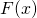is called the primitive of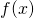: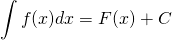wheresatisfies: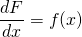f(x) F(x)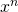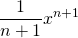a.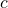(constante)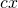b.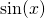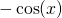c.d.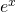e.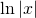f.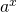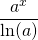g.

Furthermore the following rules hold:

h.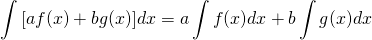Thus far the integrals are so-called indefinite integrals, meaning that the integral sign has no boundaries. This is in contrast to definite integrals which have an upper and a lower boundary.

For a definite integral we have:

i.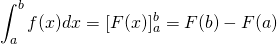In the following examples we show how we calculate integrals. The specific rule is also given.

##### Example 1

Solve:The primitive function is calculated by applying a. and thus: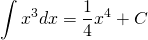##### Example 2

Solve: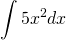The primitive is calculated by applying a. in combination with h. an thus: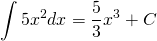##### Example 3

Solve: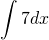The primitive is calculated by applying b. and thus: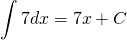##### Example 4

Solve: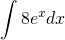The primitive is calculated by applying e. in combination met h. and thus: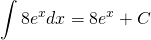##### Example 5

Solve: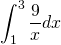The primitive is calculated by applying f. in combination with h. and i. and thus: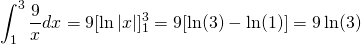Remember,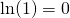.

##### Example 6

Solve: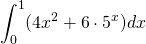The primitive is calculated by applying a. and g. in combination with h. and i. and thus: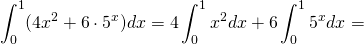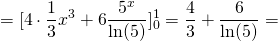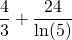##### Example 7

Solve: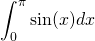The primitive is calculated by applying c. in combination with i. and thus: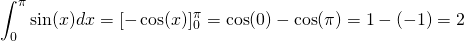##### Example 8

Solve: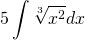This function does not seem to appear in the list of standard functions, but indirectly this is still the case. We can write:and thus rule a. can be applied with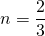.

So: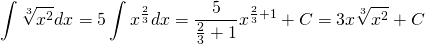##### Example 9

Solve: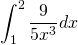This function does not seem to appear in the list of standard functions, but indirectly this is still the case. We can write: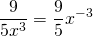and thus (by applying a., h. and i.):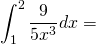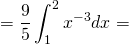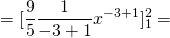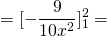=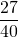##### Example 10

Solve: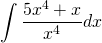This would seem an awkward integral, but the integrand (the function under the integral sign) can be written as follows: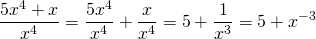and thus the integral becomes: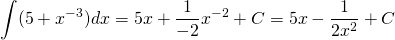Here a., b. and h. are applied.

##### Example 11

Solve: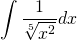We can write the integrand (the function under the integral sign):and thus the integral becomes (with a. and h.):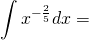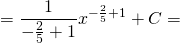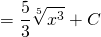0